# Physics II Electricity Magnetism Sections 23 1 to

• Slides: 94Physics II: Electricity & Magnetism Sections 23. 1 to 23. 9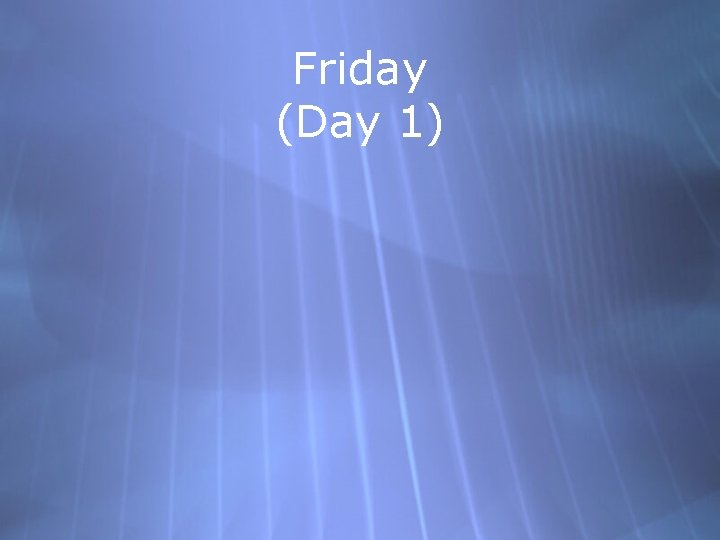Friday (Day 1)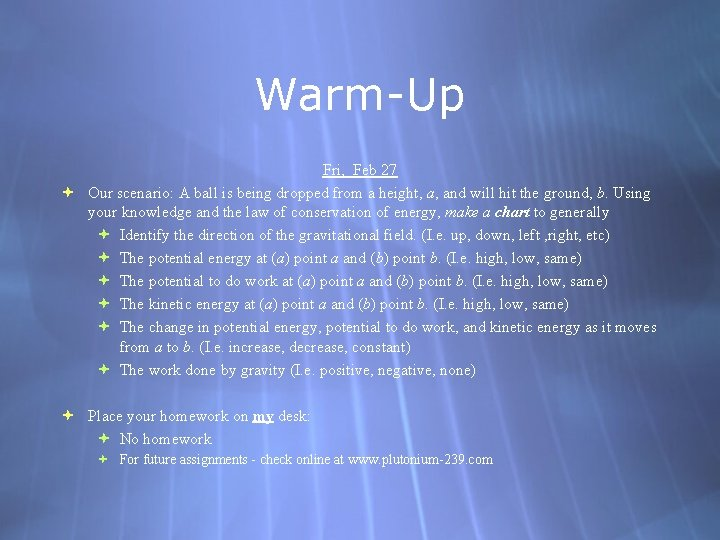Warm-Up Fri, Feb 27 Our scenario: A ball is being dropped from a height, a, and will hit the ground, b. Using your knowledge and the law of conservation of energy, make a chart to generally Identify the direction of the gravitational field. (I. e. up, down, left , right, etc) The potential energy at (a) point a and (b) point b. (I. e. high, low, same) The potential to do work at (a) point a and (b) point b. (I. e. high, low, same) The kinetic energy at (a) point a and (b) point b. (I. e. high, low, same) The change in potential energy, potential to do work, and kinetic energy as it moves from a to b. (I. e. increase, decrease, constant) The work done by gravity (I. e. positive, negative, none) Place your homework on my desk: No homework For future assignments - check online at www. plutonium-239. comScenario #1: A ball is being dropped from a height, a, and will hit the ground, b. a Direction Of Gravity b Direction Of MotionGravitational Field and Energy Using your knowledge and the law of conservation of energy, make a chart to generally Identify the direction of the gravitational field. (I. e. up, down, left , right, etc) The potential energy at (a) point a and (b) point b. (I. e. high, low, same) The potential to do work at (a) point a and (b) point b. (I. e. high, low, same) The kinetic energy at (a) point a and (b) point b. (I. e. high, low, same) The change in potential energy, potential to do work, and kinetic energy as it moves from a to b. (I. e. increase, decrease, constant) The work done by gravity (I. e. positive, negative, none) ADDITION: Write the formula’s for work, potential energy, and kinetic energy.Gravitational Field and Energy Field: y : cos = cos(0) =1 Potential Energy Potential to do Work Kinetic Energy Initial Location (a) y 0 = 0 High Low Final Location (b) Low High Change PE = PEb - PEa – Decreases, Potential = Potb - Pota – Decreases, KE = KEb - KEa + Increases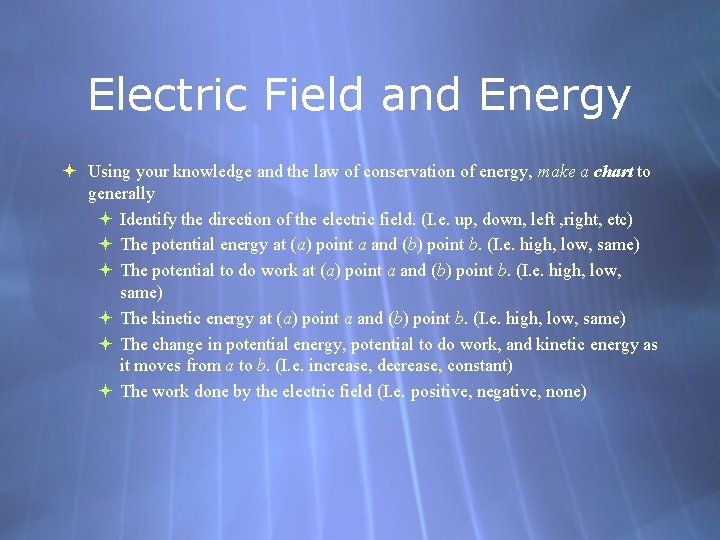Electric Field and Energy Using your knowledge and the law of conservation of energy, make a chart to generally Identify the direction of the electric field. (I. e. up, down, left , right, etc) The potential energy at (a) point a and (b) point b. (I. e. high, low, same) The potential to do work at (a) point a and (b) point b. (I. e. high, low, same) The kinetic energy at (a) point a and (b) point b. (I. e. high, low, same) The change in potential energy, potential to do work, and kinetic energy as it moves from a to b. (I. e. increase, decrease, constant) The work done by the electric field (I. e. positive, negative, none)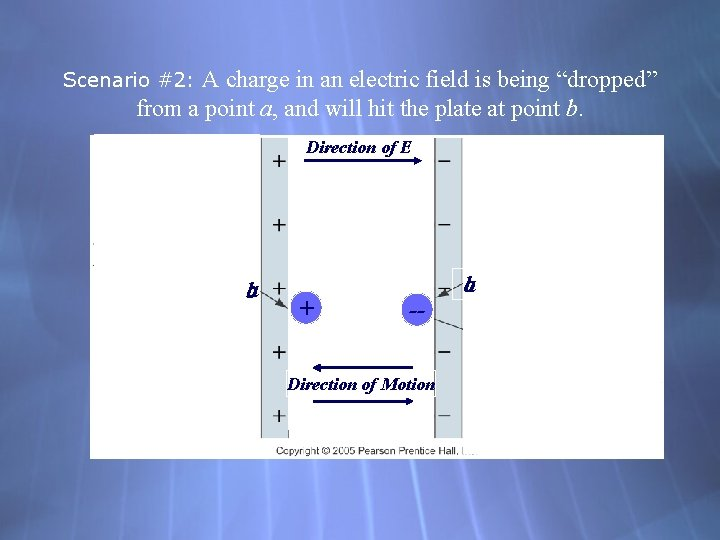A charge in an electric field is being “dropped” from a point a, and will hit the plate at point b. Scenario #2: Direction of E ba + -- Direction of Motion ab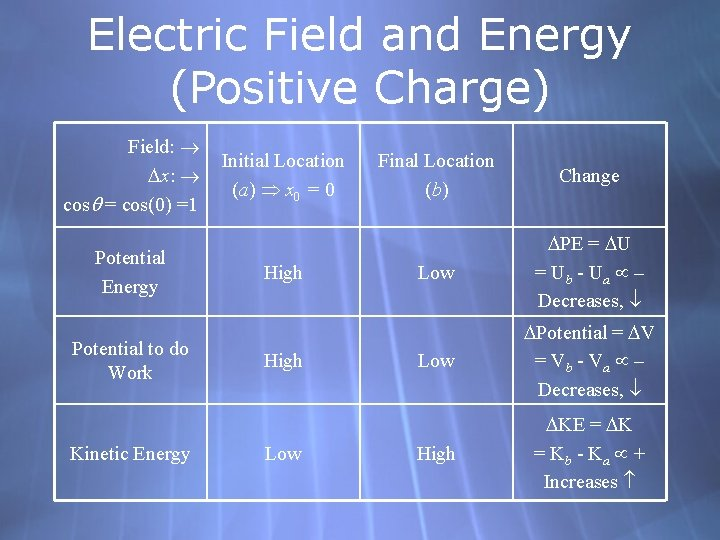Electric Field and Energy (Positive Charge) Field: x: cos = cos(0) =1 Potential Energy Potential to do Work Kinetic Energy Initial Location (a) x 0 = 0 High Low Final Location (b) Change Low PE = Ub - Ua – Decreases, Low Potential = Vb - Va – Decreases, High KE = K b - Ka + Increases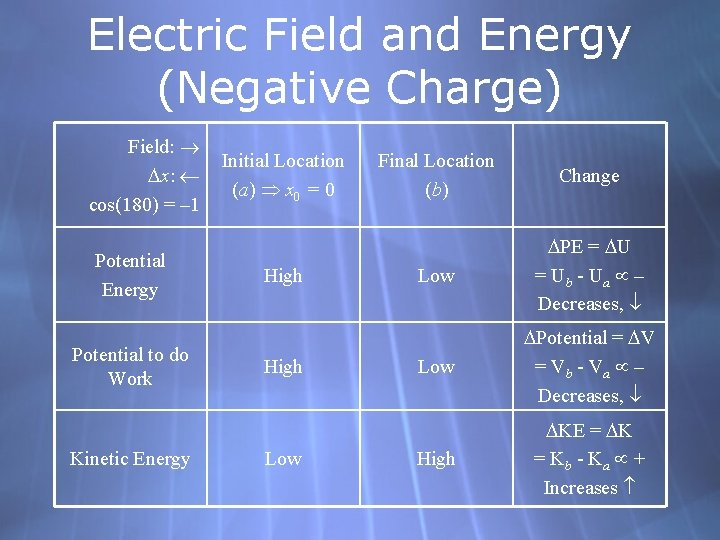Electric Field and Energy (Negative Charge) Field: x: cos(180) = – 1 Potential Energy Potential to do Work Kinetic Energy Initial Location (a) x 0 = 0 High Low Final Location (b) Change Low PE = Ub - Ua – Decreases, Low Potential = Vb - Va – Decreases, High KE = K b - Ka + Increases6 -1 Work Done by a Constant Force The work done by a constant force is defined as the distance moved multiplied by the component of the force in the direction of displacement: (6 -1)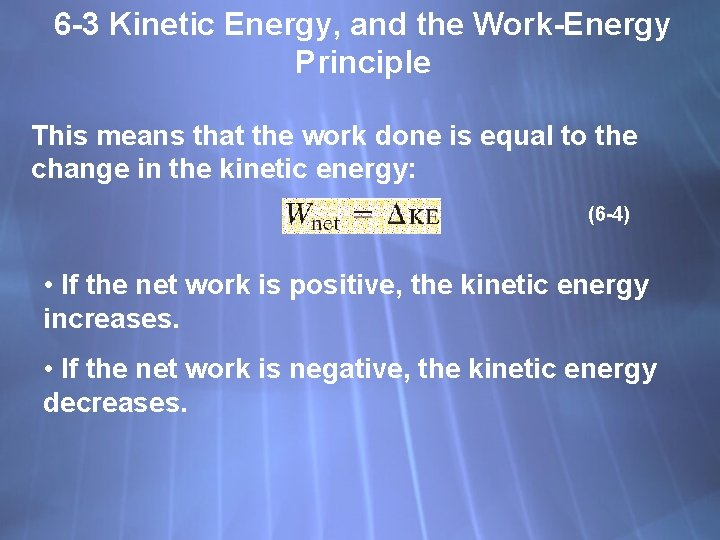6 -3 Kinetic Energy, and the Work-Energy Principle This means that the work done is equal to the change in the kinetic energy: (6 -4) • If the net work is positive, the kinetic energy increases. • If the net work is negative, the kinetic energy decreases.6 -5 Conservative and Nonconservative Forces Potential energy can only be defined for conservative forces.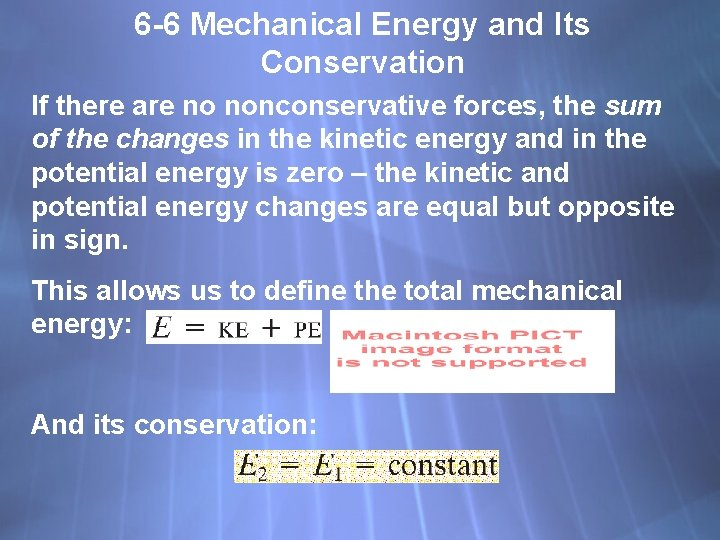6 -6 Mechanical Energy and Its Conservation If there are no nonconservative forces, the sum of the changes in the kinetic energy and in the potential energy is zero – the kinetic and potential energy changes are equal but opposite in sign. This allows us to define the total mechanical energy: And its conservation: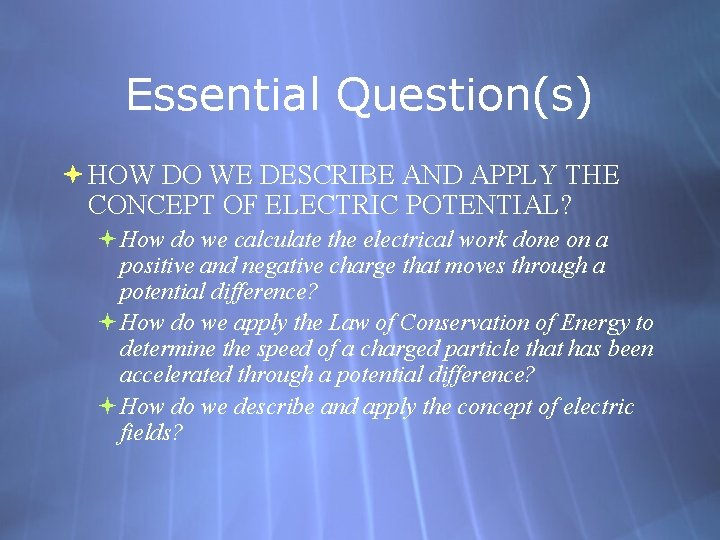Essential Question(s) HOW DO WE DESCRIBE AND APPLY THE CONCEPT OF ELECTRIC POTENTIAL? How do we calculate the electrical work done on a positive and negative charge that moves through a potential difference? How do we apply the Law of Conservation of Energy to determine the speed of a charged particle that has been accelerated through a potential difference? How do we describe and apply the concept of electric fields?Vocabulary Electric Potential Difference in Potential Difference Voltage Equipotential Lines Equipotential Surfaces Electric Dipole Moment Electron Volt Cathode Ray Tube Thermionic Emission Cathode Anode Cathode Rays Oscilloscope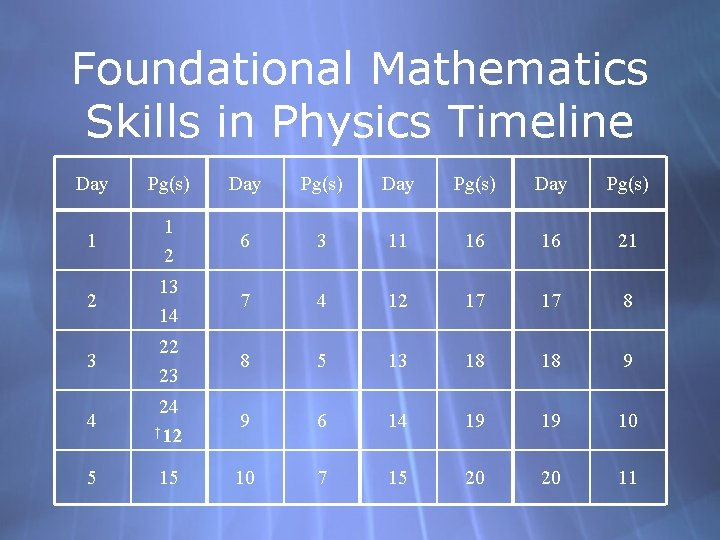Foundational Mathematics Skills in Physics Timeline Day Pg(s) 1 1 2 6 3 11 16 16 21 2 13 14 7 4 12 17 17 8 3 22 23 8 5 13 18 18 9 4 24 † 12 9 6 14 19 19 10 5 15 10 7 15 20 20 11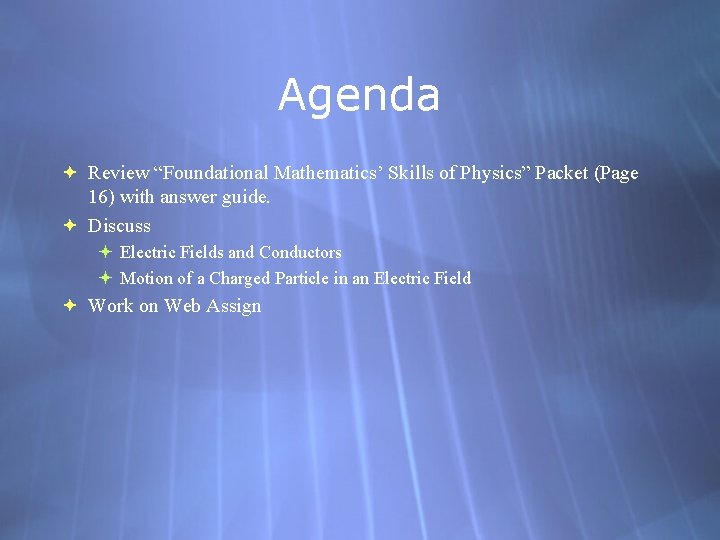Agenda Review “Foundational Mathematics’ Skills of Physics” Packet (Page 16) with answer guide. Discuss Electric Fields and Conductors Motion of a Charged Particle in an Electric Field Work on Web AssignChapter 23 Electric Potential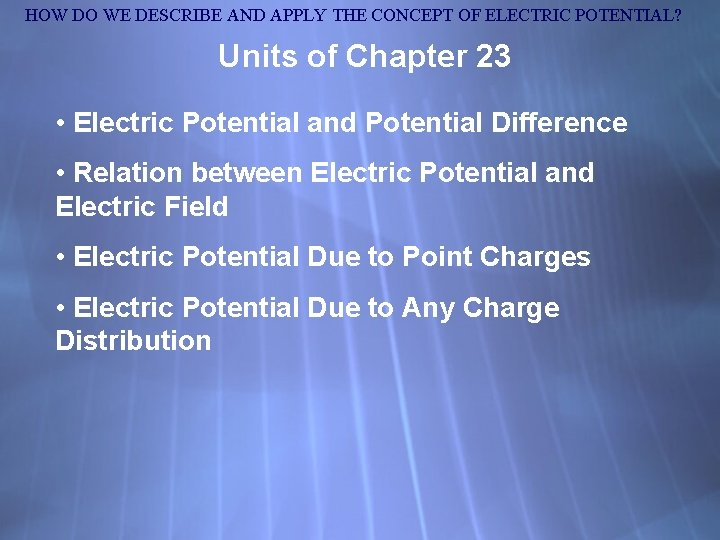HOW DO WE DESCRIBE AND APPLY THE CONCEPT OF ELECTRIC POTENTIAL? Units of Chapter 23 • Electric Potential and Potential Difference • Relation between Electric Potential and Electric Field • Electric Potential Due to Point Charges • Electric Potential Due to Any Charge Distribution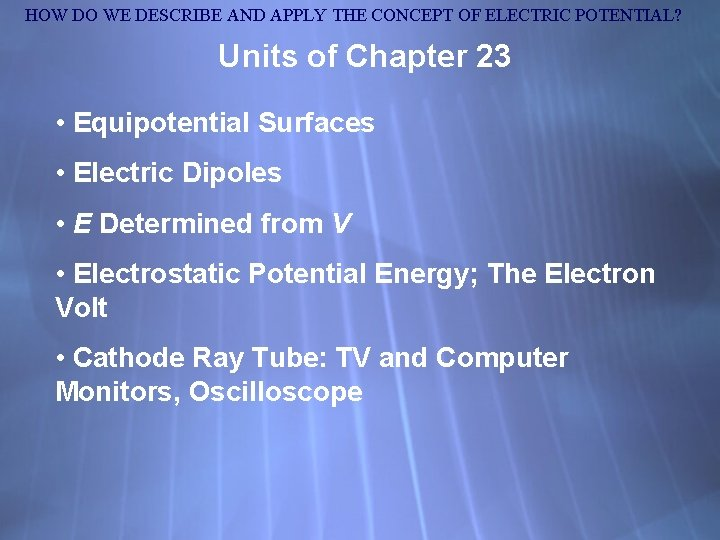HOW DO WE DESCRIBE AND APPLY THE CONCEPT OF ELECTRIC POTENTIAL? Units of Chapter 23 • Equipotential Surfaces • Electric Dipoles • E Determined from V • Electrostatic Potential Energy; The Electron Volt • Cathode Ray Tube: TV and Computer Monitors, OscilloscopeHOW DO WE DESCRIBE AND APPLY THE CONCEPT OF ELECTRIC POTENTIAL? 21. 6 The Electric Field Recall: The electric field is the force on a small charge, divided by the charge: Force on a point charge in an electric field:HOW DO WE DESCRIBE AND APPLY THE CONCEPT OF ELECTRIC POTENTIAL? Electrostatic Potential Energy and Potential Difference Analogy between gravitational and electrical potential energy:HOW DO WE DESCRIBE AND APPLY THE CONCEPT OF ELECTRIC POTENTIAL? Electrostatic Potential Energy and Potential Difference Because the electrostatic force is conservative, the potential energy can be defined. Change in electric potential energy is negative of work done by electric force: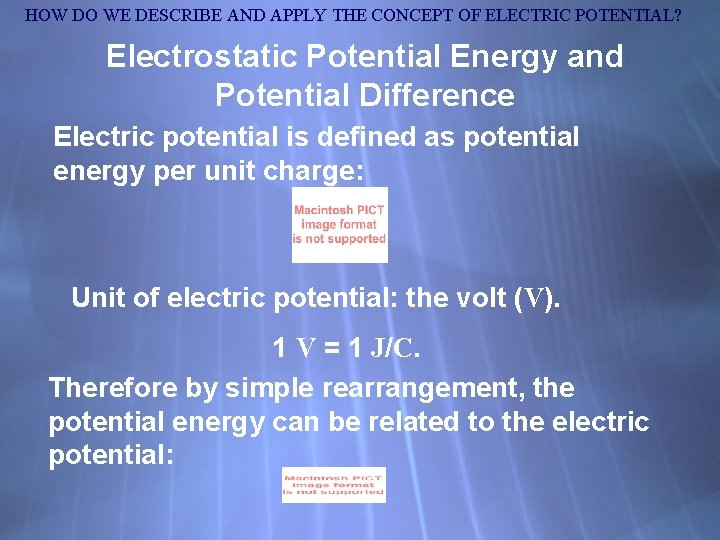HOW DO WE DESCRIBE AND APPLY THE CONCEPT OF ELECTRIC POTENTIAL? Electrostatic Potential Energy and Potential Difference Electric potential is defined as potential energy per unit charge: Unit of electric potential: the volt (V). 1 V = 1 J/C. Therefore by simple rearrangement, the potential energy can be related to the electric potential: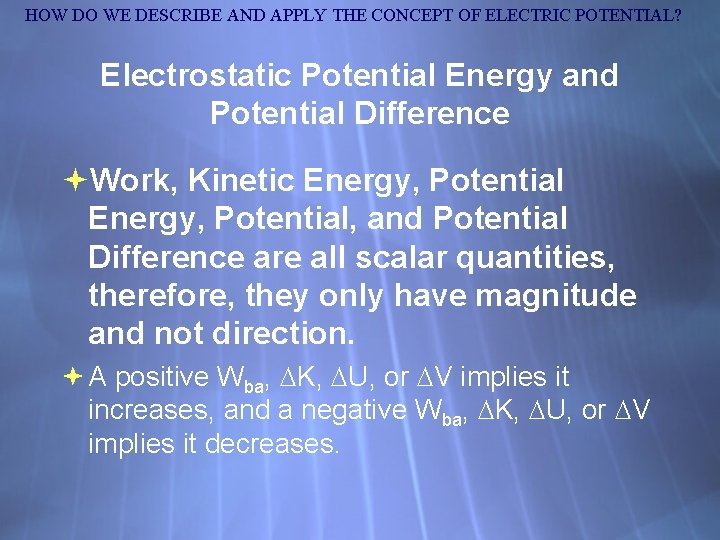HOW DO WE DESCRIBE AND APPLY THE CONCEPT OF ELECTRIC POTENTIAL? Electrostatic Potential Energy and Potential Difference Work, Kinetic Energy, Potential, and Potential Difference are all scalar quantities, therefore, they only have magnitude and not direction. A positive Wba, K, U, or V implies it increases, and a negative Wba, K, U, or V implies it decreases.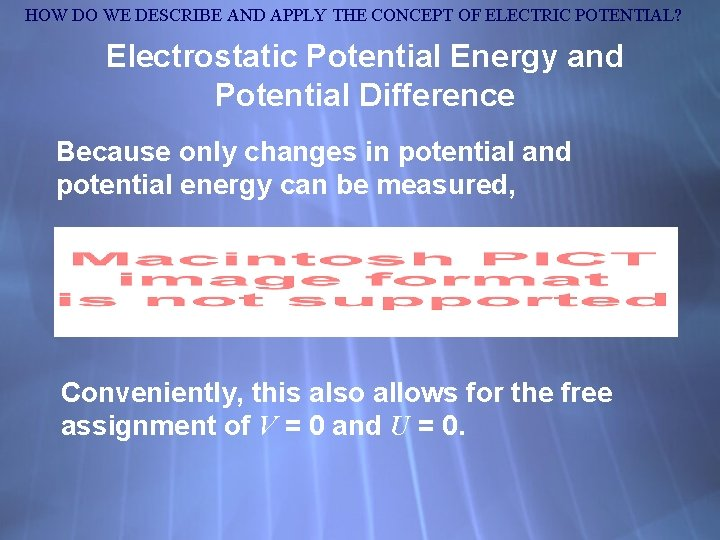HOW DO WE DESCRIBE AND APPLY THE CONCEPT OF ELECTRIC POTENTIAL? Electrostatic Potential Energy and Potential Difference Because only changes in potential and potential energy can be measured, Conveniently, this also allows for the free assignment of V = 0 and U = 0.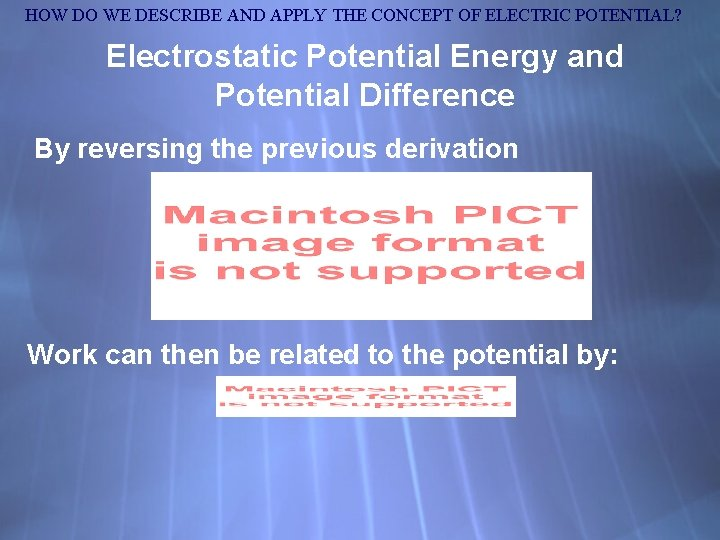HOW DO WE DESCRIBE AND APPLY THE CONCEPT OF ELECTRIC POTENTIAL? Electrostatic Potential Energy and Potential Difference By reversing the previous derivation Work can then be related to the potential by: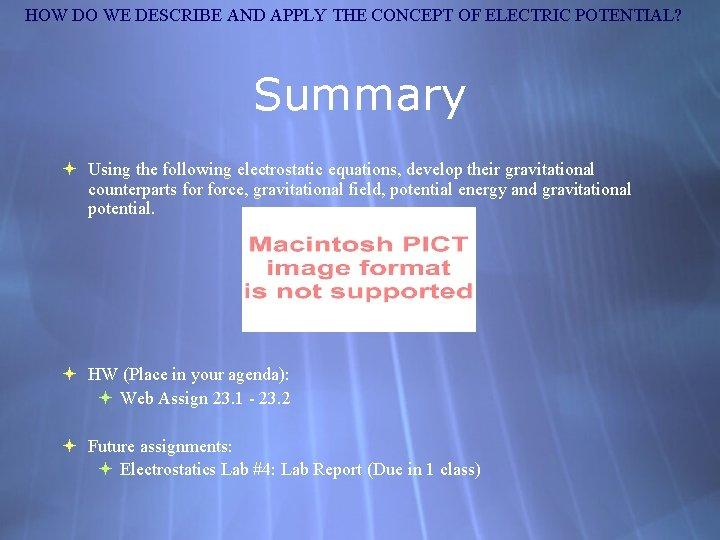HOW DO WE DESCRIBE AND APPLY THE CONCEPT OF ELECTRIC POTENTIAL? Summary Using the following electrostatic equations, develop their gravitational counterparts force, gravitational field, potential energy and gravitational potential. HW (Place in your agenda): Web Assign 23. 1 - 23. 2 Future assignments: Electrostatics Lab #4: Lab Report (Due in 1 class)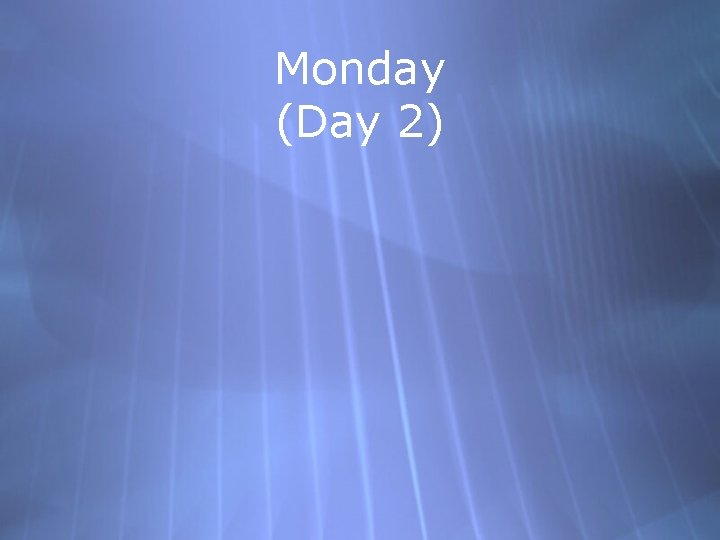Monday (Day 2)Warm-Up Mon, Mar 2 Our scenario: A positive test charge is being “dropped” in an electric field at location, a, and will hit the other plate, b. Using the following equations, derive an equation to calculate the work done by the electric field. What if the charge were negative? Place your homework on my desk: Web Assign Problems 23. 1 - 23. 2 For future assignments - check online at www. plutonium-239. comElectric Field and Energy Using your knowledge and the law of conservation of energy, make a chart to generally Identify the direction of the electric field. (I. e. up, down, left , right, etc) The potential energy at (a) point a and (b) point b. (I. e. high, low, same) The potential to do work at (a) point a and (b) point b. (I. e. high, low, same) The kinetic energy at (a) point a and (b) point b. (I. e. high, low, same) The change in potential energy, potential to do work, and kinetic energy as it moves from a to b. (I. e. increase, decrease, constant) The work done by the electric field (I. e. positive, negative, none)A charge in an electric field is being “dropped” from a point a, and will hit the plate at point b. Scenario #2: Direction of E ba + -- Direction of Motion abElectric Field and Energy (Positive Charge) Field: x: cos = cos(0) =1 Potential Energy Potential to do Work Kinetic Energy Initial Location (a) x 0 = 0 High Low Final Location (b) Change Low PE = Ub - Ua – Decreases, Low Potential = Vb - Va – Decreases, High KE = K b - Ka + IncreasesElectric Field and Energy (Negative Charge) Field: x: cos(180) = – 1 Potential Energy Potential to do Work Kinetic Energy Initial Location (a) x 0 = 0 High Low Final Location (b) Change Low PE = Ub - Ua – Decreases, Low Potential = Vb - Va – Decreases, High KE = K b - Ka + IncreasesEssential Question(s) HOW DO WE DESCRIBE AND APPLY THE CONCEPT OF ELECTRIC POTENTIAL? How do we calculate the electrical work done on a positive and negative charge that moves through a potential difference? How do we apply the Law of Conservation of Energy to determine the speed of a charged particle that has been accelerated through a potential difference? How do we describe and apply the concept of electric fields?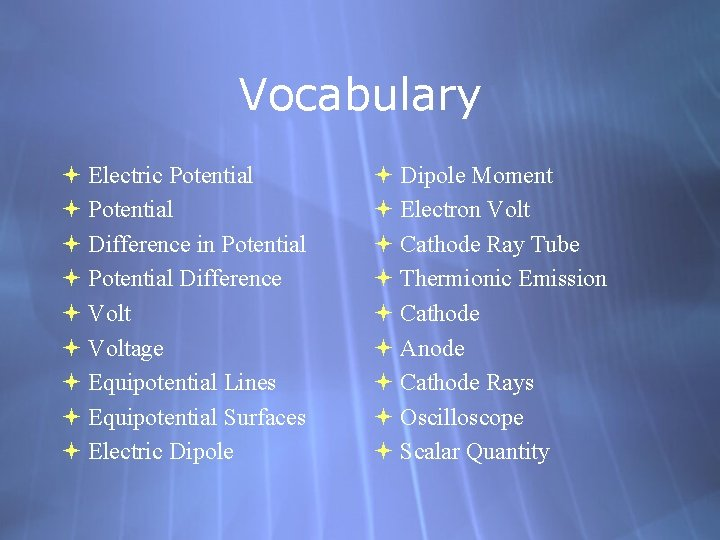Vocabulary Electric Potential Difference in Potential Difference Voltage Equipotential Lines Equipotential Surfaces Electric Dipole Moment Electron Volt Cathode Ray Tube Thermionic Emission Cathode Anode Cathode Rays Oscilloscope Scalar Quantity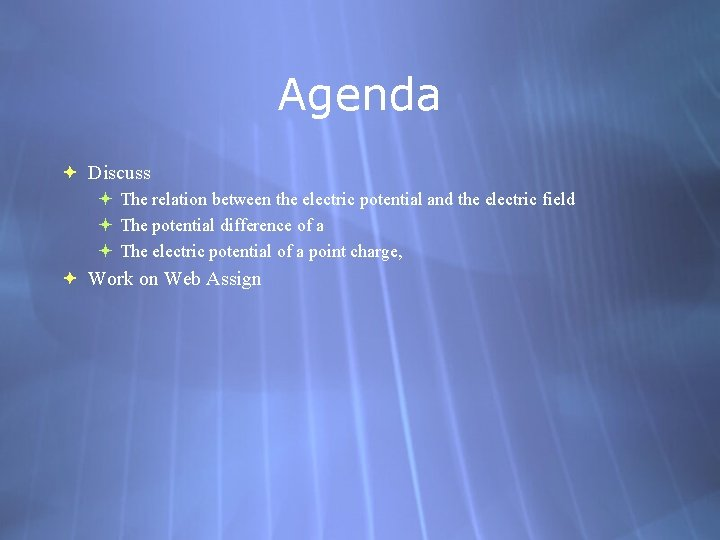Agenda Discuss The relation between the electric potential and the electric field The potential difference of a The electric potential of a point charge, Work on Web AssignHOW DO WE DESCRIBE AND APPLY THE CONCEPT OF ELECTRIC POTENTIAL? Electrostatic Potential Energy and Potential Difference Thus far, we have identified the following important equations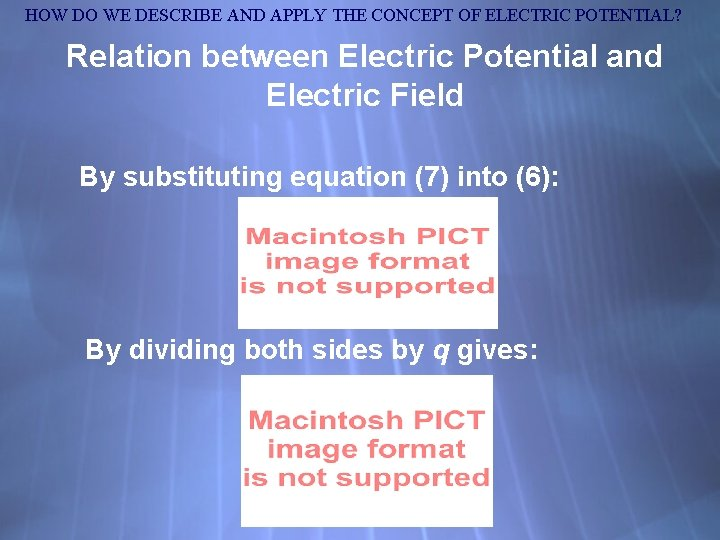HOW DO WE DESCRIBE AND APPLY THE CONCEPT OF ELECTRIC POTENTIAL? Relation between Electric Potential and Electric Field By substituting equation (7) into (6): By dividing both sides by q gives: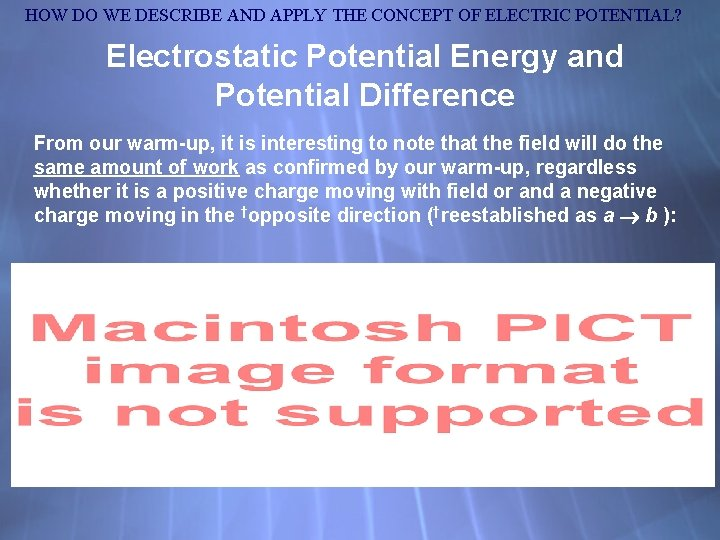HOW DO WE DESCRIBE AND APPLY THE CONCEPT OF ELECTRIC POTENTIAL? Electrostatic Potential Energy and Potential Difference From our warm-up, it is interesting to note that the field will do the same amount of work as confirmed by our warm-up, regardless whether it is a positive charge moving with field or and a negative charge moving in the †opposite direction (†reestablished as a b ): Work can then be related to the potential by: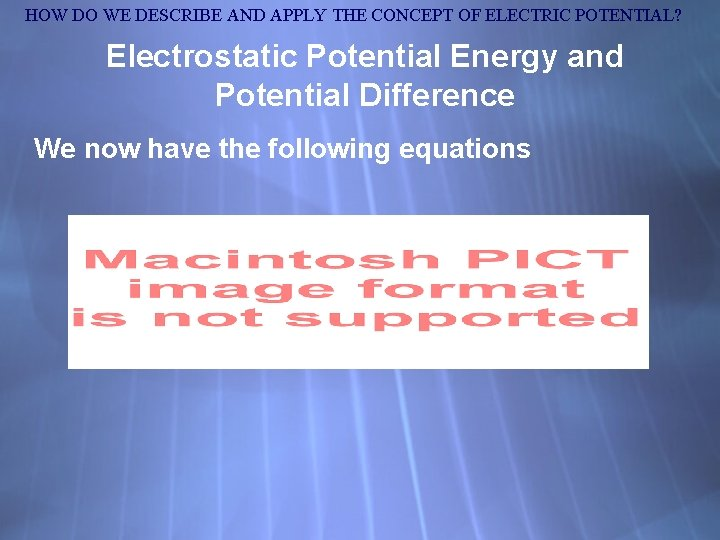HOW DO WE DESCRIBE AND APPLY THE CONCEPT OF ELECTRIC POTENTIAL? Electrostatic Potential Energy and Potential Difference We now have the following equationsHOW DO WE DESCRIBE AND APPLY THE CONCEPT OF ELECTRIC POTENTIAL? Calculating the Potential Difference, Vba, in a Uniform Field (1) Because the field and the direction of motion are the same, cos = 1, (2) Because E is uniform, it can be pulled out of the integral. (3) The negative sign implies that the potential is decreasing as it moves a distance, d, in the electric field.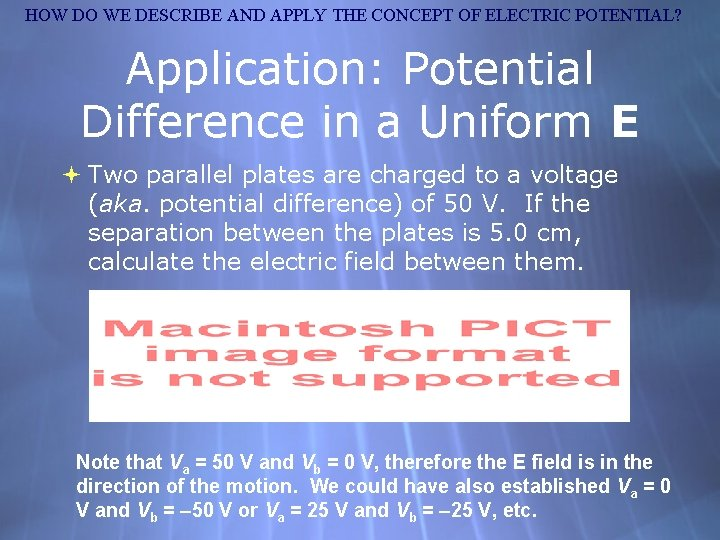HOW DO WE DESCRIBE AND APPLY THE CONCEPT OF ELECTRIC POTENTIAL? Application: Potential Difference in a Uniform E Two parallel plates are charged to a voltage (aka. potential difference) of 50 V. If the separation between the plates is 5. 0 cm, calculate the electric field between them. Note that Va = 50 V and Vb = 0 V, therefore the E field is in the direction of the motion. We could have also established Va = 0 V and Vb = – 50 V or Va = 25 V and Vb = – 25 V, etc.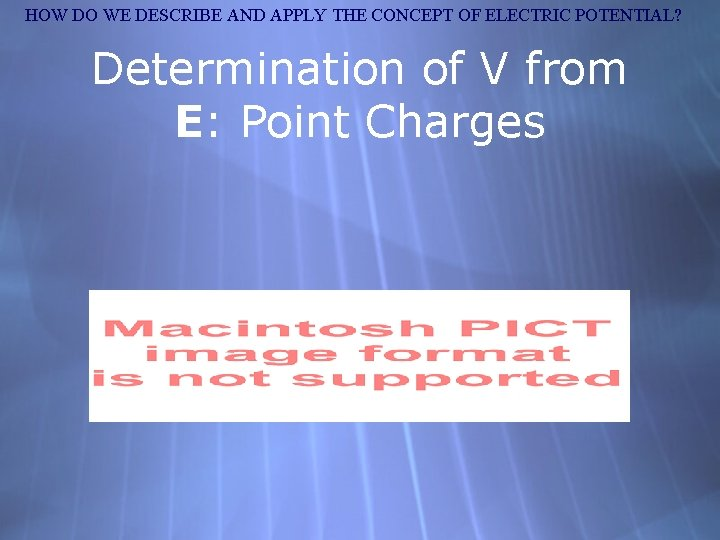HOW DO WE DESCRIBE AND APPLY THE CONCEPT OF ELECTRIC POTENTIAL? Determination of V from E: Point Charges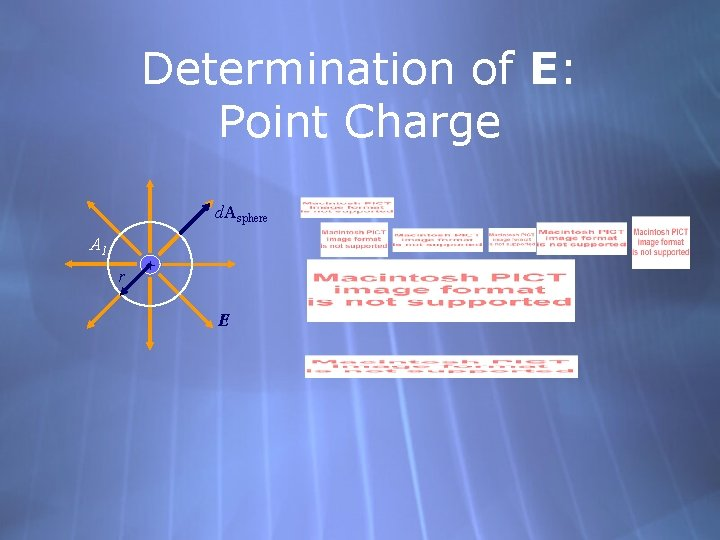Determination of E: Point Charge d. Asphere A 1 r + EHOW DO WE DESCRIBE AND APPLY THE CONCEPT OF ELECTRIC POTENTIAL? Determination of V from E: Point Charge + E dr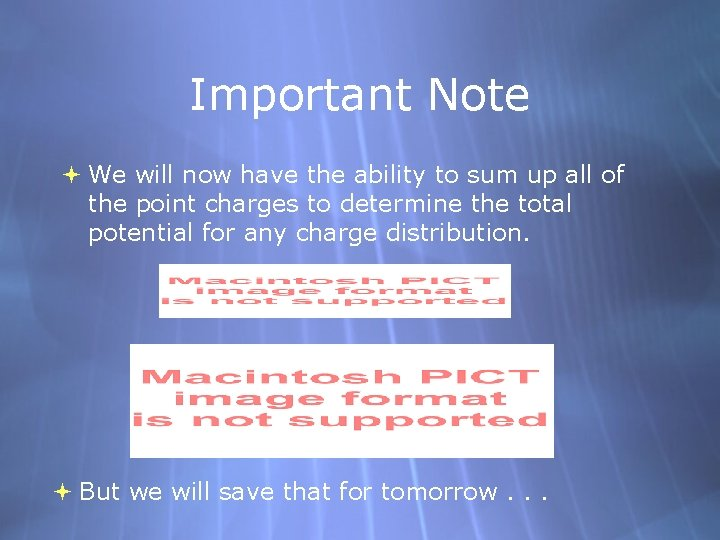Important Note We will now have the ability to sum up all of the point charges to determine the total potential for any charge distribution. But we will save that for tomorrow. . .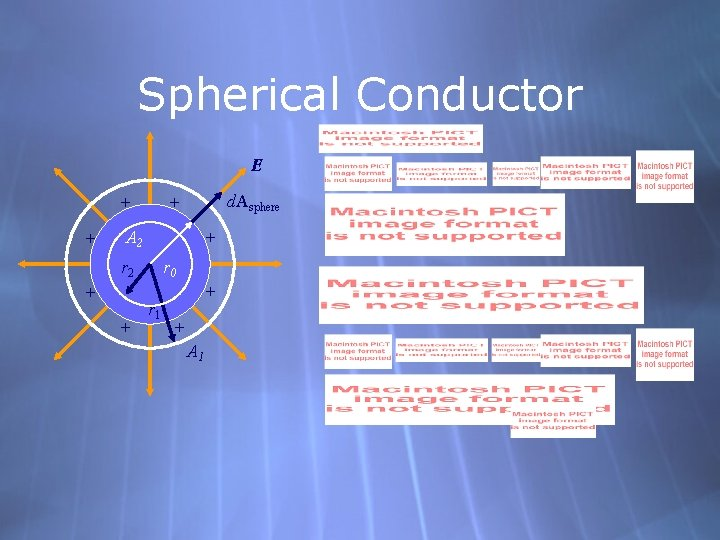Spherical Conductor E + + d. Asphere + A 2 + r 2 + + r 0 r 1 + + A 1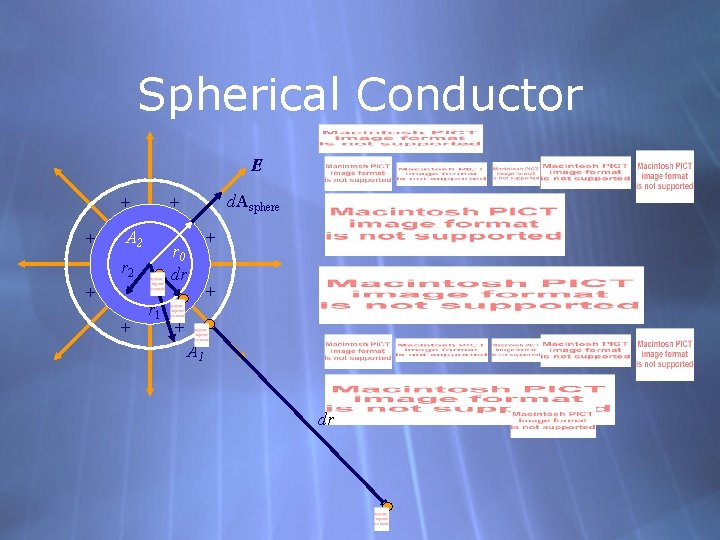Spherical Conductor E + + A 2 r 0 dr r 2 + + d. Asphere + r 1 + + + A 1 dr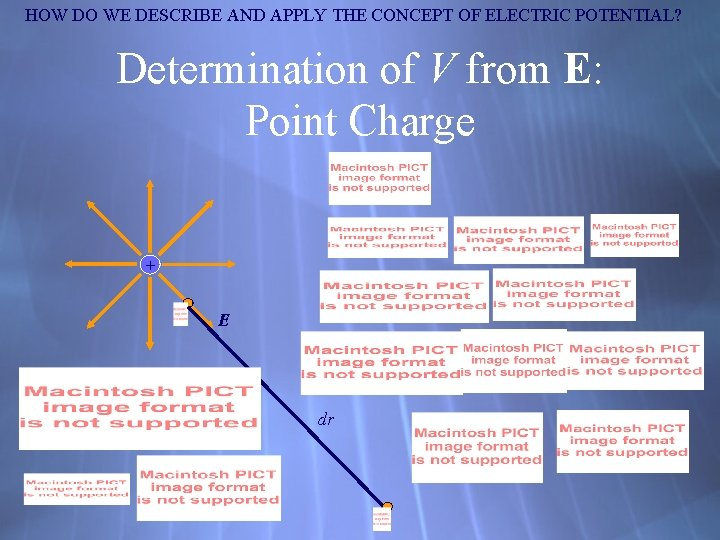HOW DO WE DESCRIBE AND APPLY THE CONCEPT OF ELECTRIC POTENTIAL? Determination of V from E: Point Charge + E dr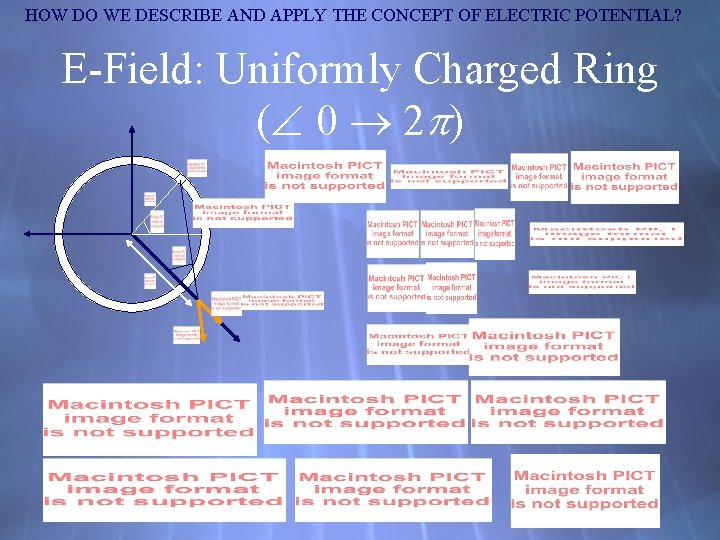HOW DO WE DESCRIBE AND APPLY THE CONCEPT OF ELECTRIC POTENTIAL? E-Field: Uniformly Charged Ring ( 0 2 )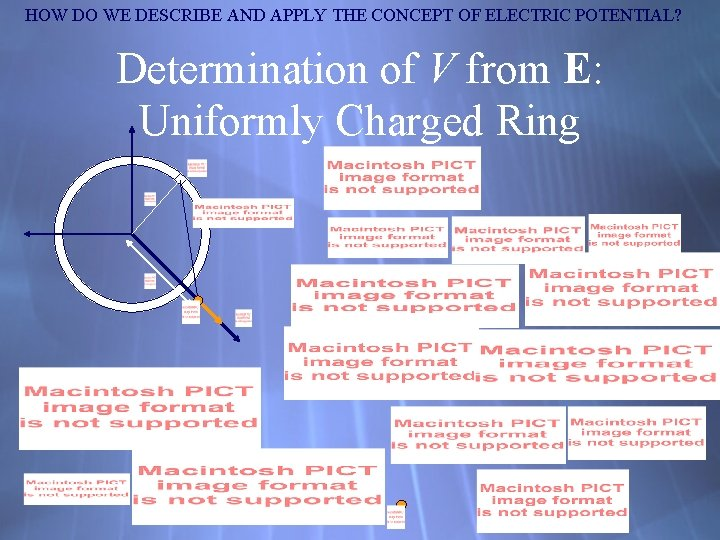HOW DO WE DESCRIBE AND APPLY THE CONCEPT OF ELECTRIC POTENTIAL? Determination of V from E: Uniformly Charged Ring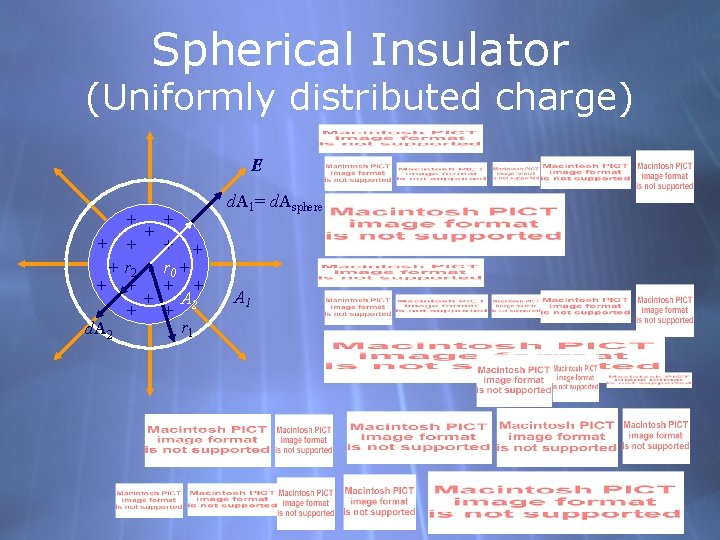Spherical Insulator (Uniformly distributed charge) E + + + + r 2 + r 0 + + + A + + 2 r 1 d. A 2 d. A 1= d. Asphere A 1HOW DO WE DESCRIBE AND APPLY THE CONCEPT OF ELECTRIC POTENTIAL? E-Field: Uniformly Charged Vertical Wire (–∞ +∞)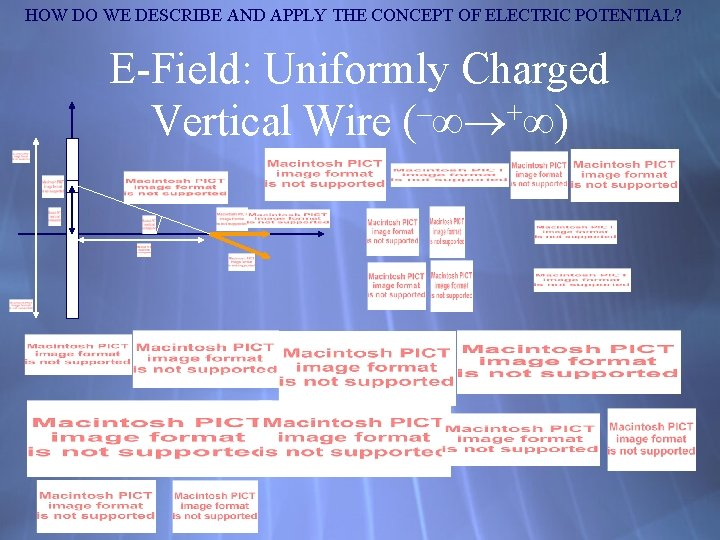HOW DO WE DESCRIBE AND APPLY THE CONCEPT OF ELECTRIC POTENTIAL? E-Field: Uniformly Charged Vertical Wire (–∞ +∞)HOW DO WE DESCRIBE AND APPLY THE CONCEPT OF ELECTRIC POTENTIAL? E-Field: Uniformly Charged Vertical Wire (–L/2 +L/2)HOW DO WE DESCRIBE AND APPLY THE CONCEPT OF ELECTRIC POTENTIAL? E-Field: †Uniformly Charged Horizontal Wire (d d+l)HOW DO WE DESCRIBE AND APPLY THE CONCEPT OF ELECTRIC POTENTIAL? E-Field: Uniformly Charged Disk (0 R)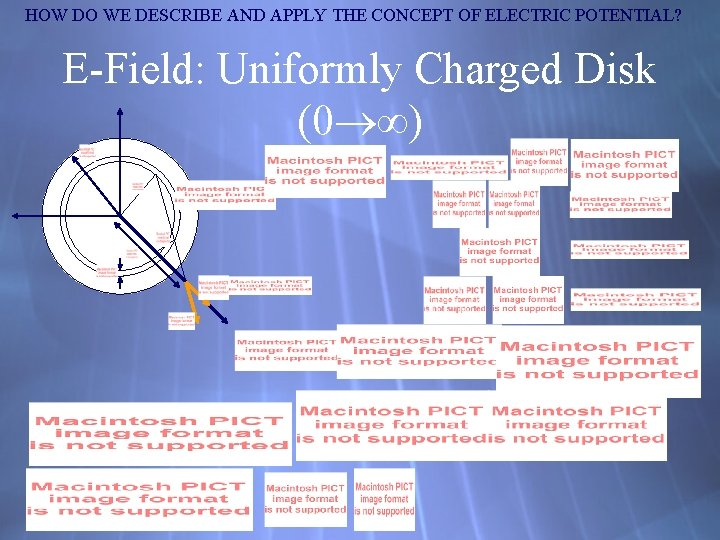HOW DO WE DESCRIBE AND APPLY THE CONCEPT OF ELECTRIC POTENTIAL? E-Field: Uniformly Charged Disk (0 ∞)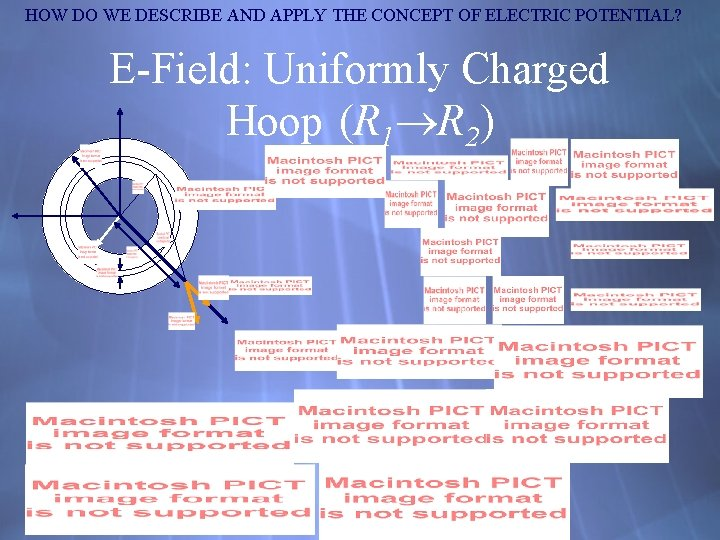HOW DO WE DESCRIBE AND APPLY THE CONCEPT OF ELECTRIC POTENTIAL? E-Field: Uniformly Charged Hoop (R 1 R 2)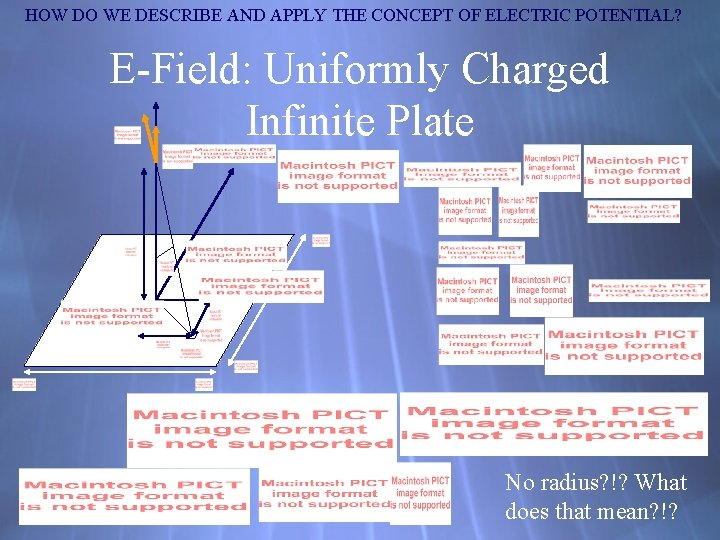HOW DO WE DESCRIBE AND APPLY THE CONCEPT OF ELECTRIC POTENTIAL? E-Field: Uniformly Charged Infinite Plate No radius? !? What does that mean? !?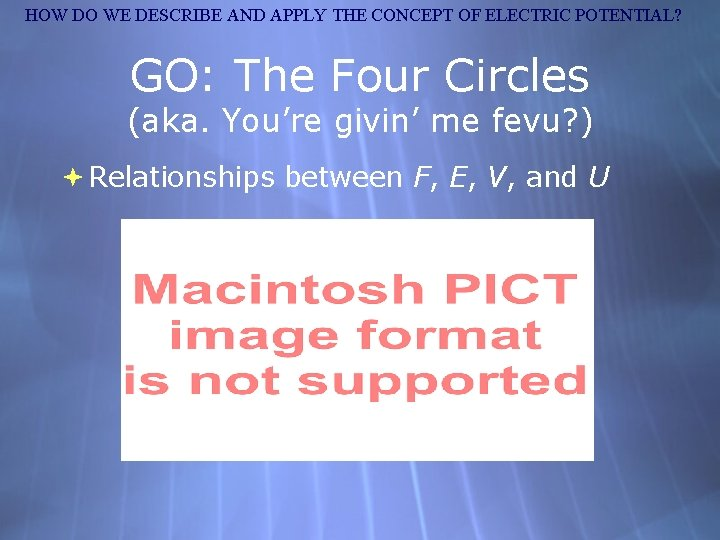HOW DO WE DESCRIBE AND APPLY THE CONCEPT OF ELECTRIC POTENTIAL? GO: The Four Circles (aka. You’re givin’ me fevu? ) Relationships between F, E, V, and U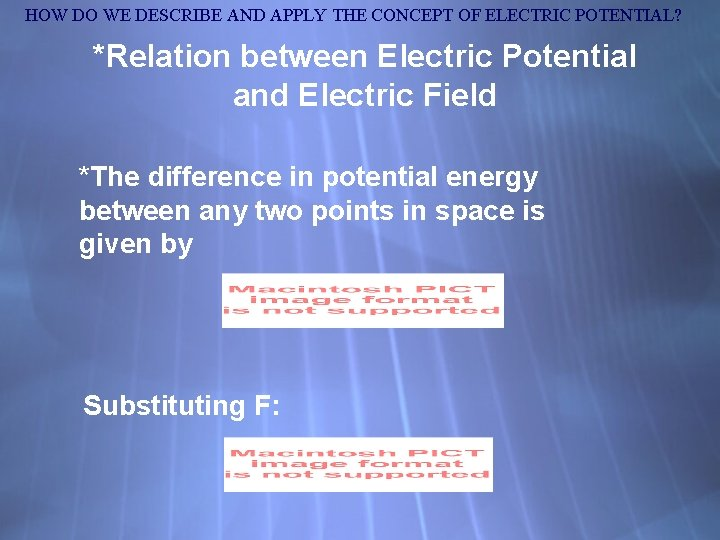HOW DO WE DESCRIBE AND APPLY THE CONCEPT OF ELECTRIC POTENTIAL? *Relation between Electric Potential and Electric Field *The difference in potential energy between any two points in space is given by Substituting F: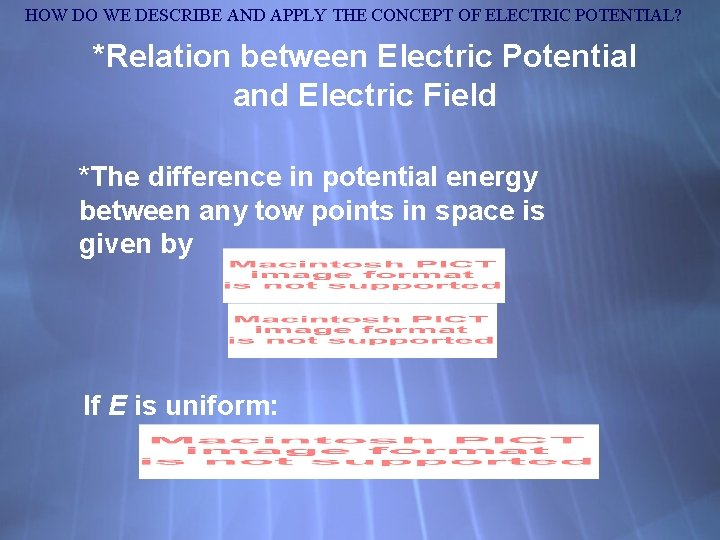HOW DO WE DESCRIBE AND APPLY THE CONCEPT OF ELECTRIC POTENTIAL? *Relation between Electric Potential and Electric Field *The difference in potential energy between any tow points in space is given by If E is uniform:HOW DO WE DESCRIBE AND APPLY THE CONCEPT OF ELECTRIC POTENTIAL? Relation between Electric Potential and Electric Field Solving for the field, If the field is not uniform, it can be calculated at multiple points: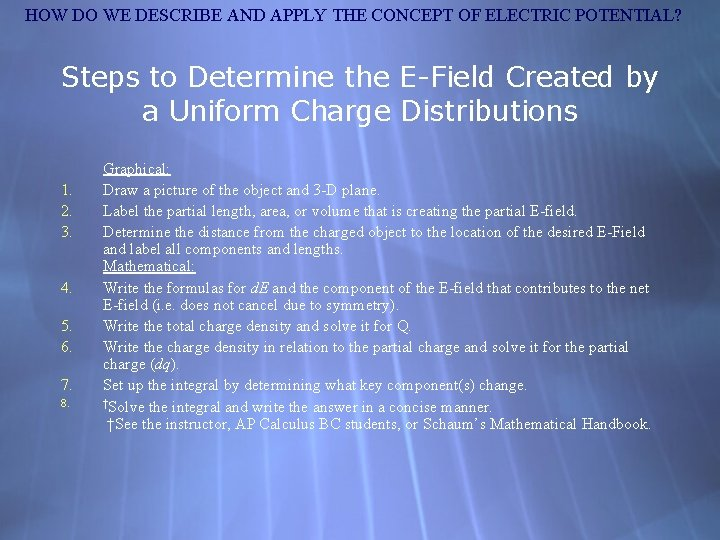HOW DO WE DESCRIBE AND APPLY THE CONCEPT OF ELECTRIC POTENTIAL? Steps to Determine the E-Field Created by a Uniform Charge Distributions 1. 2. 3. 4. 5. 6. 7. 8. Graphical: Draw a picture of the object and 3 -D plane. Label the partial length, area, or volume that is creating the partial E-field. Determine the distance from the charged object to the location of the desired E-Field and label all components and lengths. Mathematical: Write the formulas for d. E and the component of the E-field that contributes to the net E-field (i. e. does not cancel due to symmetry). Write the total charge density and solve it for Q. Write the charge density in relation to the partial charge and solve it for the partial charge (dq). Set up the integral by determining what key component(s) change. †Solve the integral and write the answer in a concise manner. †See the instructor, AP Calculus BC students, or Schaum’s Mathematical Handbook.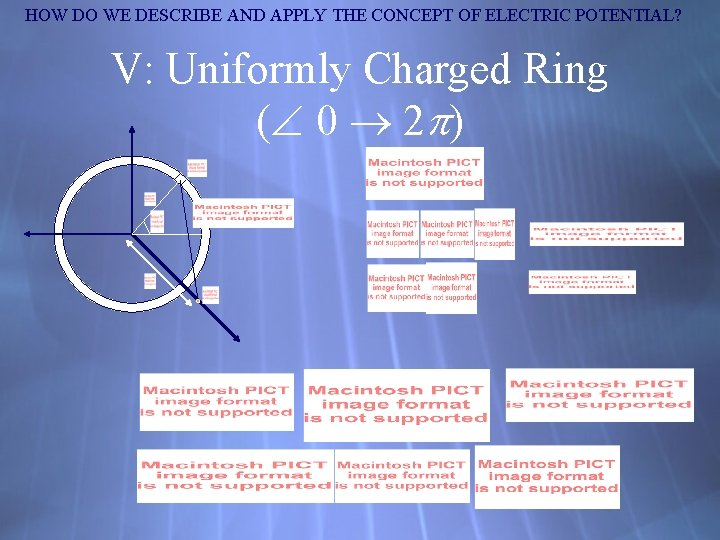HOW DO WE DESCRIBE AND APPLY THE CONCEPT OF ELECTRIC POTENTIAL? V: Uniformly Charged Ring ( 0 2 )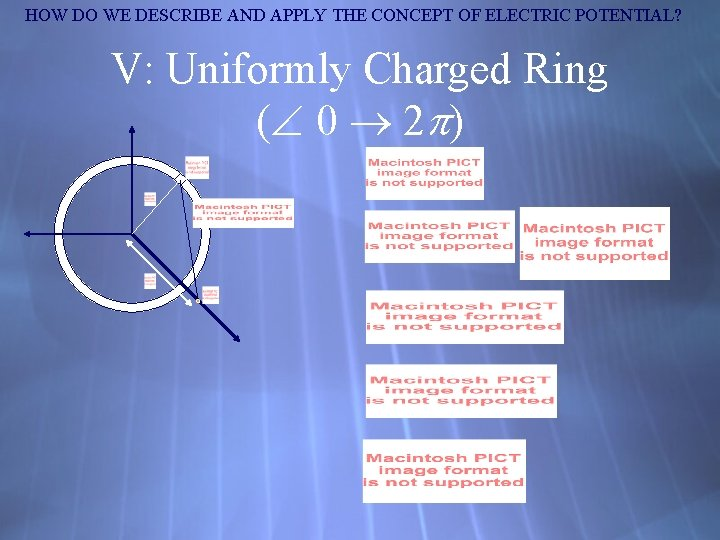HOW DO WE DESCRIBE AND APPLY THE CONCEPT OF ELECTRIC POTENTIAL? V: Uniformly Charged Ring ( 0 2 )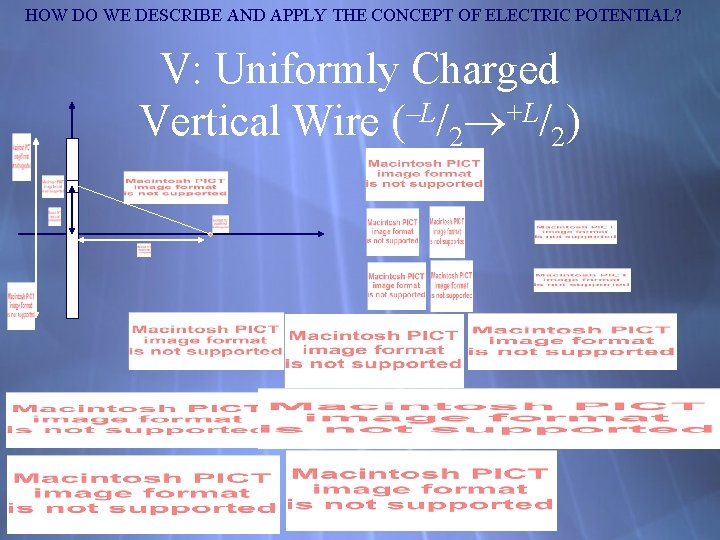HOW DO WE DESCRIBE AND APPLY THE CONCEPT OF ELECTRIC POTENTIAL? V: Uniformly Charged Vertical Wire (–L/2 +L/2)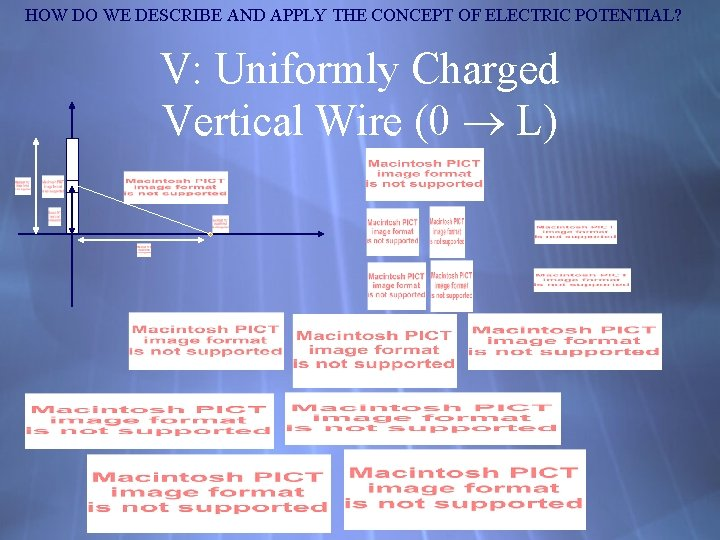HOW DO WE DESCRIBE AND APPLY THE CONCEPT OF ELECTRIC POTENTIAL? V: Uniformly Charged Vertical Wire (0 L)HOW DO WE DESCRIBE AND APPLY THE CONCEPT OF ELECTRIC POTENTIAL? V: †Uniformly Charged Horizontal Wire (d d+l)HOW DO WE DESCRIBE AND APPLY THE CONCEPT OF ELECTRIC POTENTIAL? V: Uniformly Charged Disk (0 R)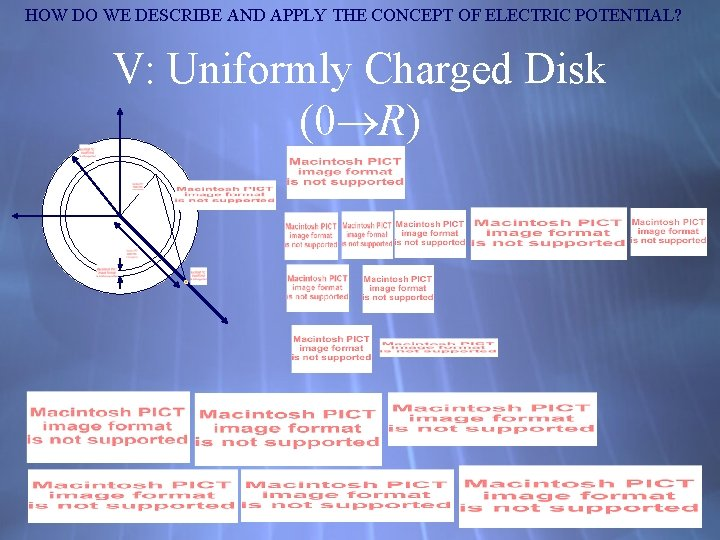HOW DO WE DESCRIBE AND APPLY THE CONCEPT OF ELECTRIC POTENTIAL? V: Uniformly Charged Disk (0 R)HOW DO WE DESCRIBE AND APPLY THE CONCEPT OF ELECTRIC POTENTIAL? V: Uniformly Charged Disk (0 R)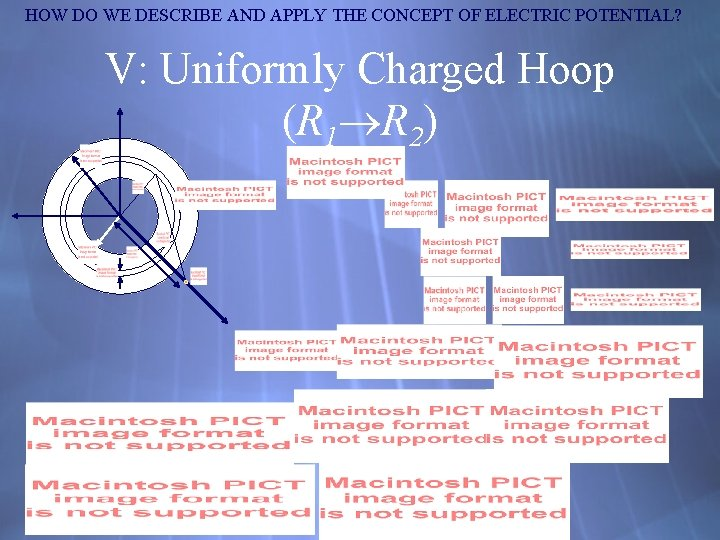HOW DO WE DESCRIBE AND APPLY THE CONCEPT OF ELECTRIC POTENTIAL? V: Uniformly Charged Hoop (R 1 R 2)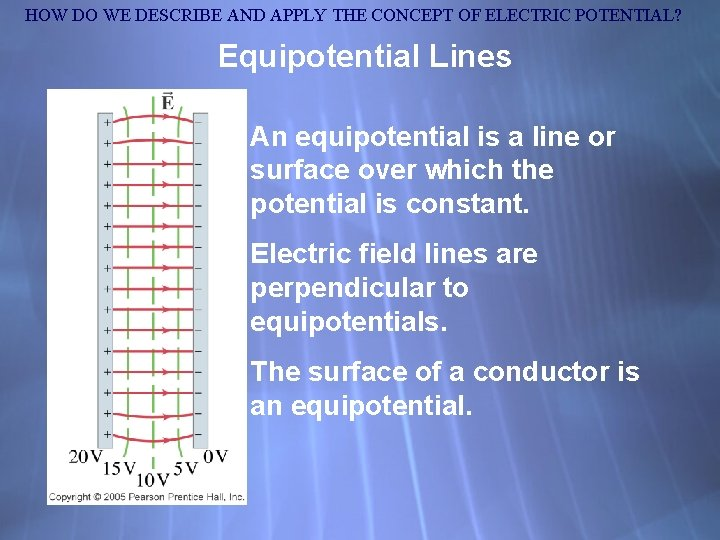HOW DO WE DESCRIBE AND APPLY THE CONCEPT OF ELECTRIC POTENTIAL? Equipotential Lines An equipotential is a line or surface over which the potential is constant. Electric field lines are perpendicular to equipotentials. The surface of a conductor is an equipotential.HOW DO WE DESCRIBE AND APPLY THE CONCEPT OF ELECTRIC POTENTIAL? Equipotential LinesHOW DO WE DESCRIBE AND APPLY THE CONCEPT OF ELECTRIC POTENTIAL? The Electron Volt, a Unit of Energy One electron volt (e. V) is the energy gained by an electron moving through a potential difference of one volt.HOW DO WE DESCRIBE AND APPLY THE CONCEPT OF ELECTRIC POTENTIAL? Electric Potential Due to Point Charges The electric potential due to a point charge can be derived using calculus.HOW DO WE DESCRIBE AND APPLY THE CONCEPT OF ELECTRIC POTENTIAL? Things to add EM Field 6: Equipotential Lines and Potentials Values (i. e. Work done = 0 V) Graphic Organizers + Finish Chapter 21 GO’s (i. e. 4 squares) Derivations (for V integrals) Plot of E and V vs. r for point charge & nonconducting sphere) Electric Dipoles (Derivation - See sheet)HOW DO WE DESCRIBE AND APPLY THE CONCEPT OF ELECTRIC POTENTIAL? Electric Potential Due to Point Charges These plots show the potential due to (a) positive and (b) negative charge.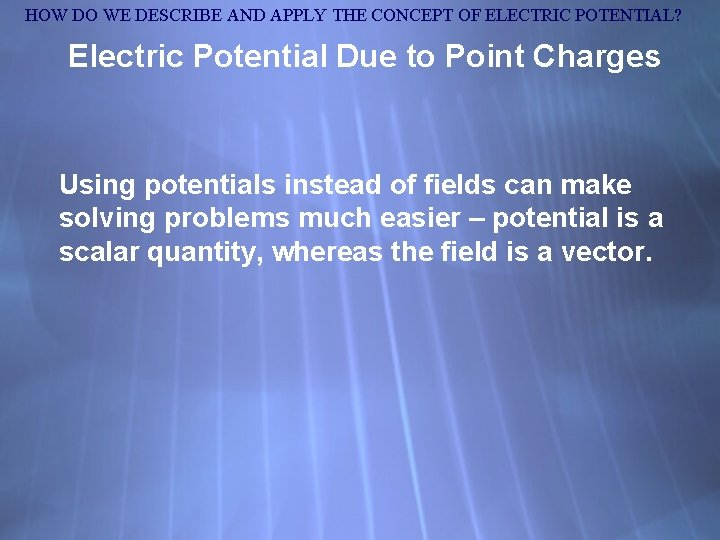HOW DO WE DESCRIBE AND APPLY THE CONCEPT OF ELECTRIC POTENTIAL? Electric Potential Due to Point Charges Using potentials instead of fields can make solving problems much easier – potential is a scalar quantity, whereas the field is a vector.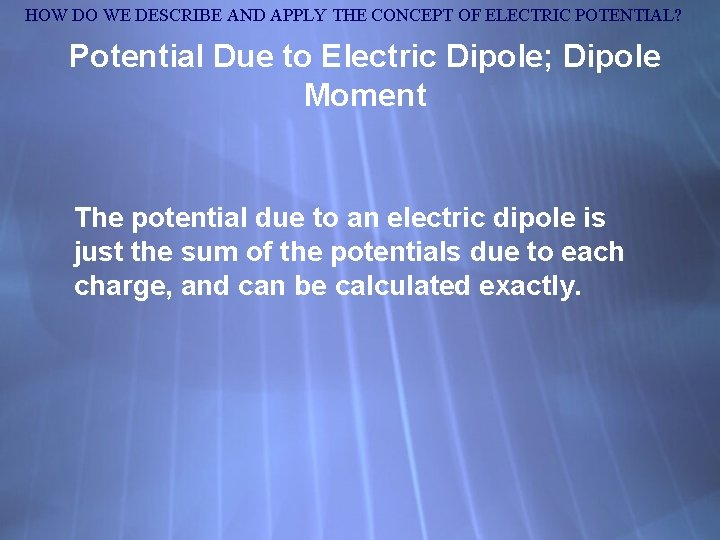HOW DO WE DESCRIBE AND APPLY THE CONCEPT OF ELECTRIC POTENTIAL? Potential Due to Electric Dipole; Dipole Moment The potential due to an electric dipole is just the sum of the potentials due to each charge, and can be calculated exactly.HOW DO WE DESCRIBE AND APPLY THE CONCEPT OF ELECTRIC POTENTIAL? Potential Due to Electric Dipole; Dipole Moment Approximation for potential far from dipole: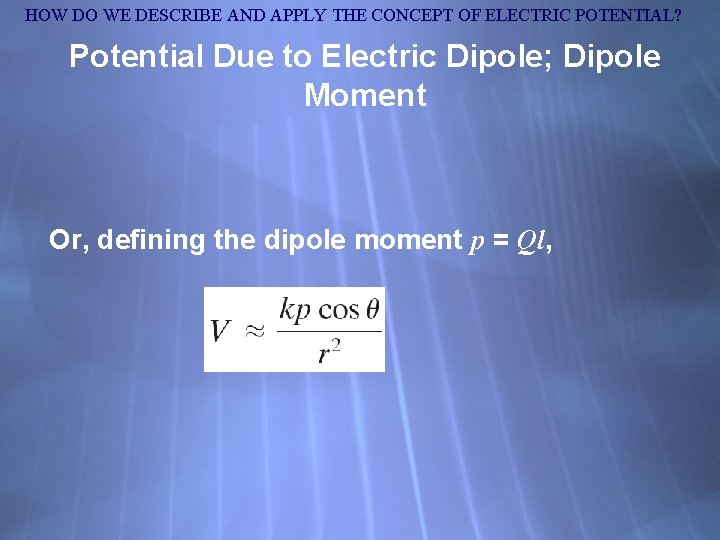HOW DO WE DESCRIBE AND APPLY THE CONCEPT OF ELECTRIC POTENTIAL? Potential Due to Electric Dipole; Dipole Moment Or, defining the dipole moment p = Ql,HOW DO WE DESCRIBE AND APPLY THE CONCEPT OF ELECTRIC POTENTIAL? Cathode Ray Tube: TV and Computer Monitors, Oscilloscope A cathode ray tube contains a wire cathode that, when heated, emits electrons. A voltage source causes the electrons to travel to the anode.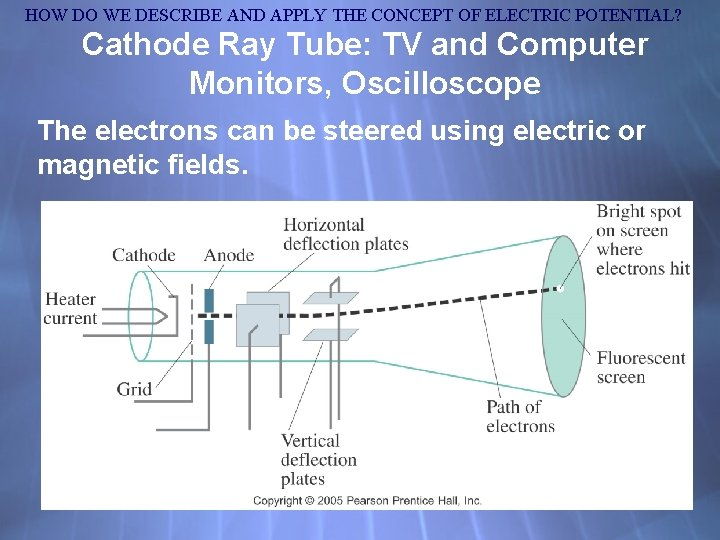HOW DO WE DESCRIBE AND APPLY THE CONCEPT OF ELECTRIC POTENTIAL? Cathode Ray Tube: TV and Computer Monitors, Oscilloscope The electrons can be steered using electric or magnetic fields.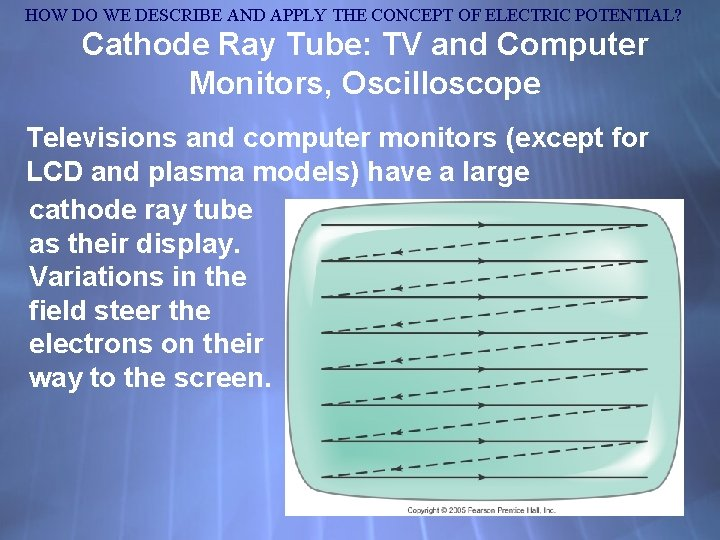HOW DO WE DESCRIBE AND APPLY THE CONCEPT OF ELECTRIC POTENTIAL? Cathode Ray Tube: TV and Computer Monitors, Oscilloscope Televisions and computer monitors (except for LCD and plasma models) have a large cathode ray tube as their display. Variations in the field steer the electrons on their way to the screen.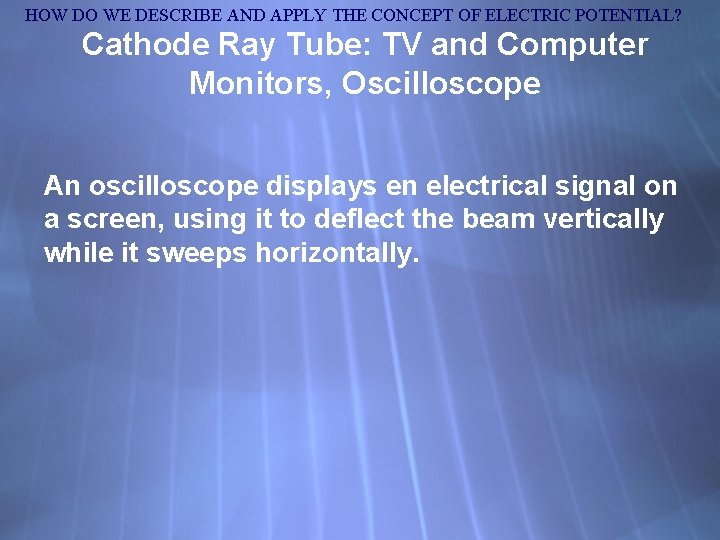HOW DO WE DESCRIBE AND APPLY THE CONCEPT OF ELECTRIC POTENTIAL? Cathode Ray Tube: TV and Computer Monitors, Oscilloscope An oscilloscope displays en electrical signal on a screen, using it to deflect the beam vertically while it sweeps horizontally.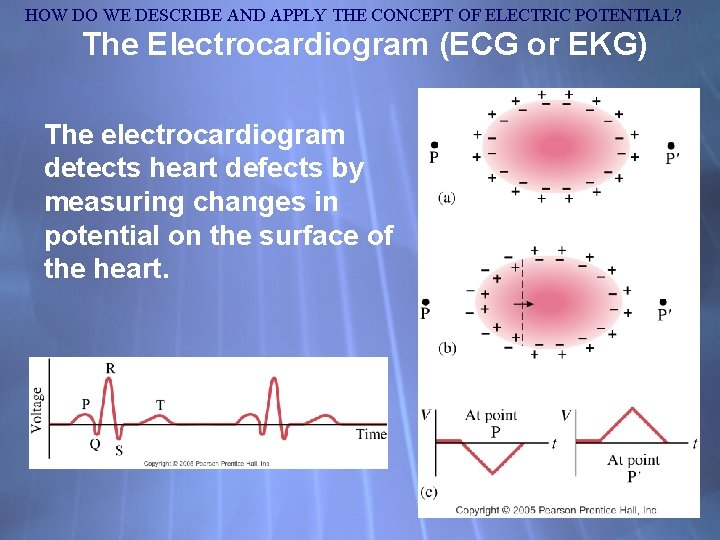HOW DO WE DESCRIBE AND APPLY THE CONCEPT OF ELECTRIC POTENTIAL? The Electrocardiogram (ECG or EKG) The electrocardiogram detects heart defects by measuring changes in potential on the surface of the heart.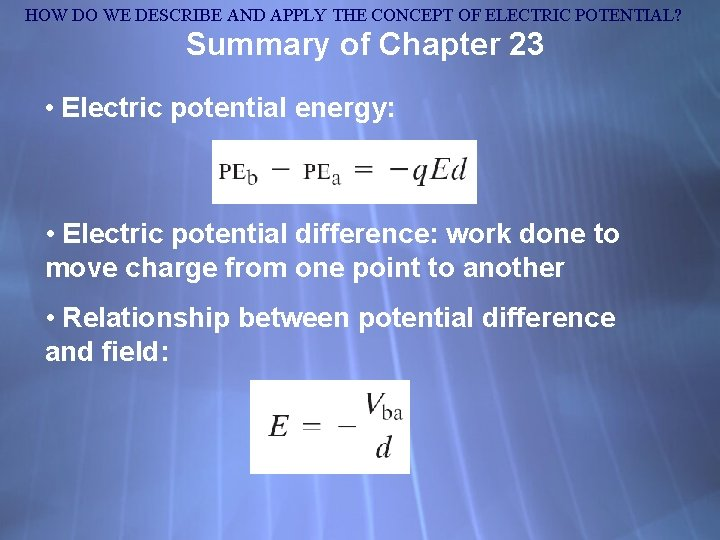HOW DO WE DESCRIBE AND APPLY THE CONCEPT OF ELECTRIC POTENTIAL? Summary of Chapter 23 • Electric potential energy: • Electric potential difference: work done to move charge from one point to another • Relationship between potential difference and field: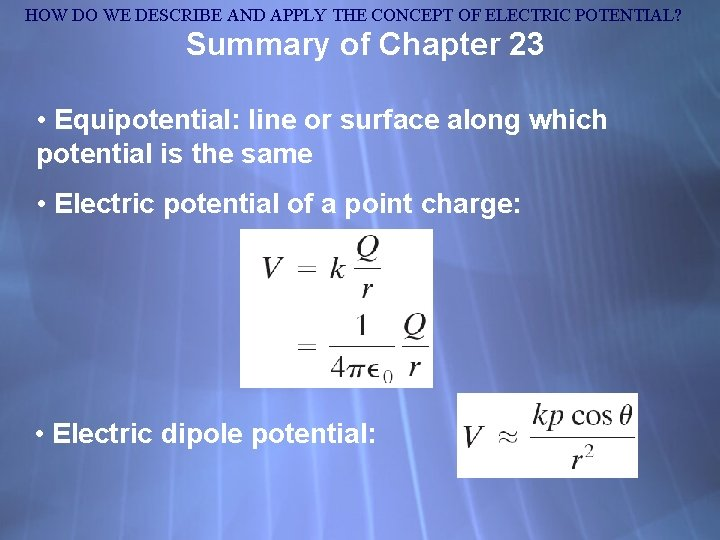HOW DO WE DESCRIBE AND APPLY THE CONCEPT OF ELECTRIC POTENTIAL? Summary of Chapter 23 • Equipotential: line or surface along which potential is the same • Electric potential of a point charge: • Electric dipole potential: